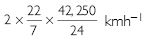# NCERT Solutions for Class 9 Science Chapter 8 - Motion

##### Question 1:

An object has moved through a distance. Can it have zero displacement? If yes, support your answer with an example.

Yes, a body can have zero displacement even if the distance covered is not zero.
Example: Let a body moves round a complete circle once, the distance covered by body is 2pr, yet its displacement is zero because it has come back to the starting point.

##### Question 2:

A farmer moves along the boundary of a square field of side 10 m in 40 s. What will be the magnitude of displacement of the farmer at the end of 2 minute 20 seconds from his initial position?

Total time taken = 2 min 20 s = 140 s
In 40 s, he goes once round the square starting from A. In 140 s, he will complete 140/40 = 3.5 rounds. If he starts from A, he will reach point C after 3.5 rounds.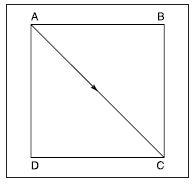Net displacement is AC ==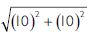=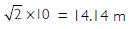##### Question 3:

Which of the following is true for displacement?
(a) It cannot be zero.
(b) Its magnitude is greater than the distance travelled by an object.

Both (a) and (b) are wrong.

##### Question 4:

Distinguish between speed and velocity.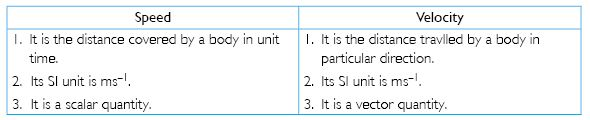##### Question 5:

Under what condition(s) is the magnitude of average velocity of an object is equal to its average speed?

The magnitude of average velocity is equal to the magnitude of average speed only if the body is moving in a straight line.

##### Question 6:

What does the odometer of an automobile measure?

An odometer measures the distance covered by an automobile.

##### Question 7:

What does the path of an object look like when it is in uniform motion?

The path during uniform rectilinear motion is along a straight line. However, a particle may move in uniform motion along a circular path where its speed is uniform.

##### Question 8:

During an experiment, a signal from a spaceship reached the ground station in 5 minutes. What was the distance of spaceship from the ground station? The signal travels at the speed of light, i.e., 3 x 108 ms-1.

Time (t) = 5 min = 5 x 60 s = 300 s,
Distance = Speed x Time
= 3 x 108 ms-1 x 300 s
= 9 x 1010 m

##### Question 9:

When will you say a body is in (i) uniform acceleration? (ii) non-uniform acceleration?

(i) Acceleration of a body is said to be uniform if its velocity changes by equal amounts in equal intervals of time, however small these intervals may be.
(ii) Acceleration of a body is said to be non-uniform if its velocity changes by unequal amounts in equal intervals of time.

##### Question 10:

A bus decreases its speed from 80 kmh-1 to 60 kmh-1 in 5 s. Find the acceleration of the bus.

u = 80 kmh-1; v = 60 kmh-1; t = 5

u =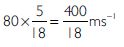v =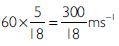Acceleration, a =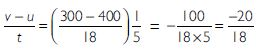(-) ve sign shows that this is retardation.

\ Retardation, - a =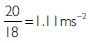##### Question 11:

A train starting from a railway station and moving with uniform acceleration attains a speed 40 kmh-1 in 10 minutes. Find its acceleration.

u = 0 ms-1; v =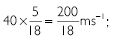t = 10 min = 10 x 60 = 600s

Therefore, a =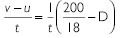a =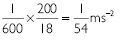##### Question 12:

What is the nature of the distance-time graphs for uniform and non-uniform motion of an object?

The distance-time graph in case of uniform motion is a straight line as shown in Fig. (a) and in case of non-uniform motion, the graph is a curved line as shown in Fig. (b).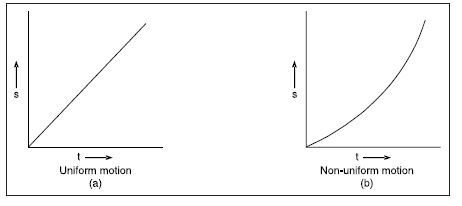##### Question 13:

What can you say about the motion of an object whose distance-time graph is a straight line parallel to the time axis?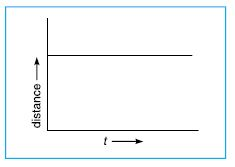The body is at rest.
[Since, its distance from a reference point remains the same.]

##### Question 14:

What can you say about the motion of an object if its speed-time graph is a straight line parallel to time axis?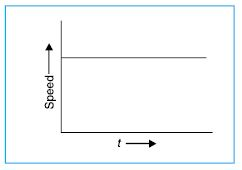It indicates that the body is moving with uniform speed.
[Since, its speed does not change with time.]

##### Question 15:

What is the quantity which is measured by the area occupied below the velocity-time graph?

The area between velocity-time graph and time axis gives the distance covered.

##### Question 16:

A bus starting from rest moves with a uniform acceleration of 0.1 ms-2 for 2 minutes. Find (a) the speed acquired (b) the distance travelled.

u = 0; a = 0.1 ms-2; t = 2 min = 120 s; v = ?; s = ?
Using v = u + at, we have:
v = 0 + 0.1 x 120
v = 12 ms-1

Using s =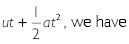s = 0 x 120 + 1/2 x 0.1 (120)2 =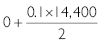s = 720 m

##### Question 17:

A train is travelling at a speed of 90 kmh-1. Brakes are applied so as to produce a uniform acceleration of -0.5 ms-2. Find how far the train will go before it is brought to rest.

u = 90 kmh-1 =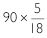.ms-1 = 25 ms-1; v = 0 ms-1; a = - 0.5 ms-1; s = ?

Using v2 - u2 = 2as, we have:

s =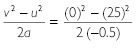s = 625 m

##### Question 18:

A trolley while going down an inclined plane has an acceleration of 2 cms-2. What will be its velocity 3 s after the start?

u = 0; a = 2 cms-2; v = ? ; t = 3 s
Using v = u + at, we have,
v = 0 + 2 (3)
v = 6 cms-1

##### Question 19:

A racing car has a uniform acceleration of 4 ms-2. What distance will it cover in 10 s after start?

u = 0; t = 10 s; a = 4 ms-2; s =?

Using s =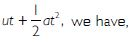s =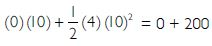s = 200 m

##### Question 20:

A stone is thrown in vertically upward direction with a velocity of 5 ms-1. If the acceleration of the stone during its motion is 10 ms-2 in the downward direction, what will be the height attained by the stone and how much time will it take to reach there?

u = 5 ms-1; v = 0 [at highest point];
a = - 10 ms-2; s = h = ?; t = ?
Using v2 - u2 = 2ah, we have,
(0)2 - (5)2 = 2 (- 10) h
or -25 = -20 h
or h = 1.25 m
Using v = u + at, we have,
0 = 5 - 10 t
or 10 t = 5
t = 0.5 s

##### Question 21:

An athlete completes one round of circular track of diameter 200 m in 40 s. What will be the distance covered and the displacement at the end of 2 minutes 20 s?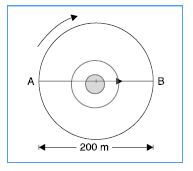Total time = 2 min 20 s
= 120 + 20 = 140 s

Since athlete completes one round in 40 s, it will complete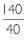= 3.5 rounds in 140 s.

Distance covered = 3.5 × pD

=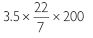= 2,200 m
A particle starting from A in clockwise direction returns to A in 3 rounds and reaches B in next half round.
\ Displacement in 3.5 rounds = AB = 200 m

##### Question 22:

Joseph jogs from one end A to the other end B of a straight 300 m road in 2 minutes 30 seconds and then turns around and jogs 100 m back to point C in another 1 minute. What are Joseph’s average speeds and velocities in jogging (a) from A to B and (b) from A to C?

Time taken to cover from A to B = 2 min 30 s
= 2 x 60 + 30 = 150 s
Time taken from B to C = 1 min = 60 s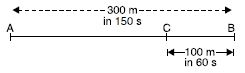Fig.
Total distance covered = AB + BC = 300 + 100 = 400 m
Total time = 150 + 60 = 210 s

(a) Average speed between A and B =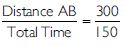= 2 ms-1

Average velocity between A and B =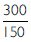= 2 m s-1 from A to B

(b) Average speed between A and C =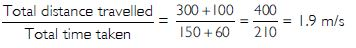Average velocity between A and C =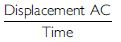=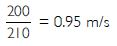##### Question 23:

Abdul, while driving to school, computes the average speed for his trip to be 20 kmh-1. On his return trip along the same route, there is less traffic and the average speed is 30 kmh-1. What is the average speed for Abdul’s trip?

Let the distance of Abdul’s school be S and t1 is the time taken by Abdul to reach school.

t1 =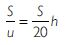and t2 =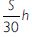. (t2 is time taken by Abdul to reach back home)

Total distance of full trip = S + S = 2S

Total time t1 + t2 =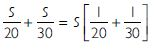t =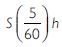Average speed for trip =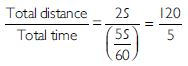= 24 kmh-1

##### Question 24:

A motorboat starting from rest on a lake accelerates in a straight line at a constant rate of 3.0 ms-2 for 8.0 s. How far does the boat travel during this time?

u = 0; a = 3 ms-2; t = 8 s; s = ?

Using s =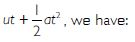s =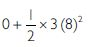s = 96 m

##### Question 25:

A driver of a car travelling at 52 kmh-1 applies the brakes and accelerates uniformly in opposite direction. The car stops in 5 s. Another driver going at 3 kmh-1 applies his brakes slowly and stops in 10 s. On the same graph paper, plot the speed versus time graph for the two cars. Which of the two cars travelled farther after the brakes were applied?

The required graph is as shown in the figure below.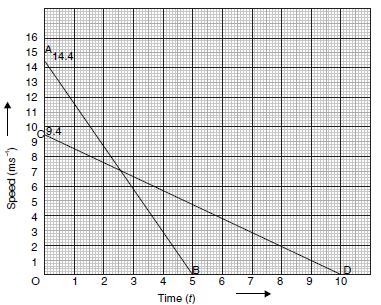Initial speed for 1st car = 52 kmh-1

=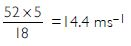Initial speed for 2nd car = 34 kmh-1

=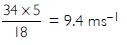Final speed for both cars = 0
Time taken by 1st car to stop = 5 s
Time taken by 2nd car to stop = 10 s
Corresponding to these values, the speed-time graph is as shown in the figure.
Distance travelled by 1st car = Area of triangle OAB

=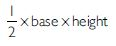= ½ x OB x OA

=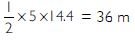Similarly, distance travelled by 2nd car = Area of triangle OCD = ½ x OD x OC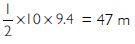Thus, the 2nd car has travelled more distance than the 1st car.

##### Question 26:

The following figure shows the distance-time graph of three objects A, B and C. Study the graph and answer the following questions: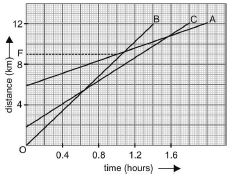(a) Which of the three objects is travelling the fastest?
(b) Are the three ever at the same point on the road?
(c) How far has C travelled when B passes A?
(d) How far has B travelled by the time it passes C?

(a) The slope of distance-time graph gives the speed and that of B is the maximum. Hence, B is the fastest.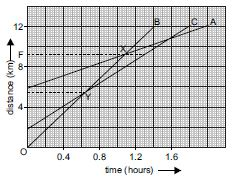(b) All the three lines do not intersect at a single point; therefore, all are never at the same point.
(c) B passes A at X, C is at F i.e., at 9.3 km.
(d) B passes C at Y after covering 5 km.

##### Question 27:

A ball is gently dropped from a height of 20 m. If its velocity increases uniformly at the rate of 10 ms-2, with what velocity will it strike the ground? After what time will it strike the ground?

u = 0; s = 20 m; a = 10 ms-2; v =? ; t = ?
Using v2 - u2 = 2as, we have
v2 - (0)2 = 2 × 10 × 20 = 400
v = 20 ms-1

Using s =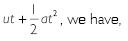20 =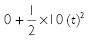t2 = 4
or t = 2 s

##### Question 28:

The speed-time graph for a car is shown in the figure.
(a) Find how far the car travels in first 4 seconds. Shade the area on the graph that represents the distance travelled by the car during the period.
(b) Which part of the graph represents uniform motion of the car?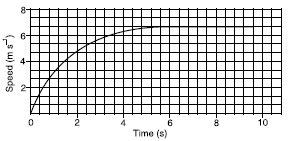(a) The shaded area gives the distance covered in first four seconds.
On x-axis 5 squares = 2s

1 square =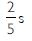On y-axis 3 squares = 2 ms-1

1 square =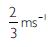Area of 1 square =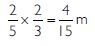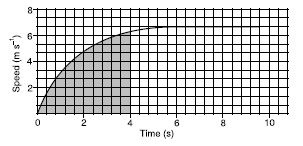This area represents distance.
No. of squares up to t = 4 s are 63

Distance covered in first 4 s =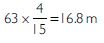(b) The straight line path from t = 6 s to t = 10 s represents uniform motion.

##### Question 29:

State which of the following situations are possible and give an example for each of these:
(a) An object with a constant acceleration but with zero velocity.
(b) An object moving with an acceleration but with uniform speed.
(c) An object moving in a certain direction with an acceleration in the perpendicular direction.

All the three situations are possible.
Examples:
(a) When an object is projected upwards, its velocity at top-most point is zero although it has an acceleration of 9.8 m s-2 in the downward direction.
(b) When a body is moving in a circular path with uniform speed, its acceleration (towards centre of the circle) is perpendicular to the direction of motion (which is tangential to circular path).
(c) Motion of the bob of a pendulum has instantaneous velocity zero but acceleration towards the centre which is in the perpendicular direction.

##### Question 30:

An artificial satellite is moving in a circular orbit of radius 42,250 km. Calculate its speed if it takes 24 hours to revolve around the earth.

Speed =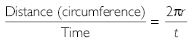=